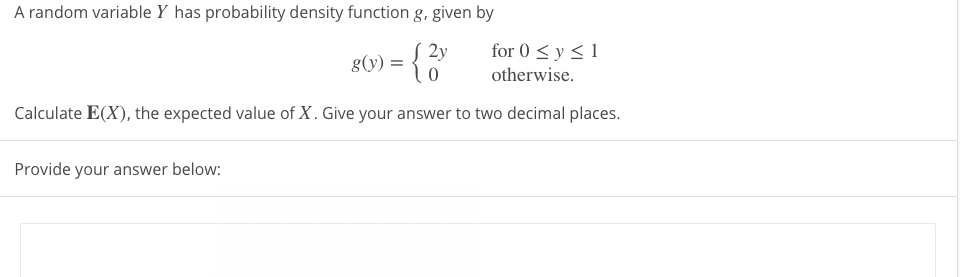# A random variable Y has probability density function g, given by for 0 < y < 1 8(v) = { 2y g(y) = otherwise. Calculate E(X), the expected value of X. Give your answer to two decimal places.

Questionhelp_outlineImage TranscriptioncloseA random variable Y has probability density function g, given by for 0 < y < 1 8(v) = { 2y g(y) = otherwise. Calculate E(X), the expected value of X. Give your answer to two decimal places. fullscreen

1 Rating

### Want to see this answer and more?

Experts are waiting 24/7 to provide step-by-step solutions in as fast as 30 minutes!*

*Response times may vary by subject and question complexity. Median response time is 34 minutes for paid subscribers and may be longer for promotional offers.
Tagged in
Math
Calculus

### Integration